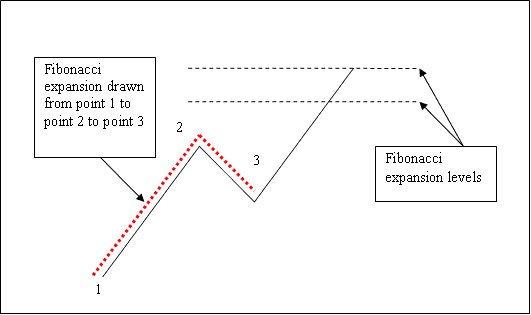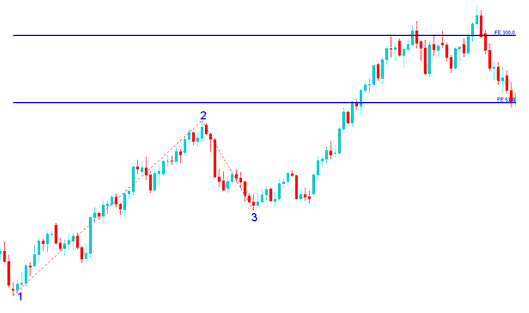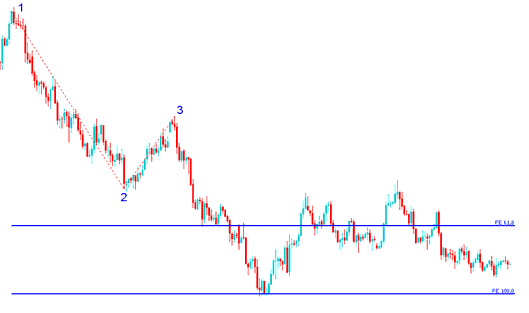# Fibonacci Projection Strategies in Indices Trading

## What is Fibonacci Projection in Indices Trading?

Fibonacci projection is drawn using 3 chart points.

To draw Fibonacci Projection levels we wait until the stock indexes price retracement is complete and the stock indexes price starts to move in the original direction of the Indices trend. Where the retracement reaches is used as chart point 3.

The Fibonacci projection example explained and illustrated below shows the 3 Chart Points where the Fibonacci projection indicator is drawn, marked as Chart point 1, 2 and 3. Chart point 1 is where the indices trend started, Chart point 2 is where the indices trend pulled back and retraced and Chart point 3 is where the stock indices retracement reached as shown on the Fibonacci Projection Tool example explained and illustrated below.Indices Trade With Fibonacci Projection? Fibonacci Projection Strategy using Fibonacci Projection Levels

Please note where these Fibonacci Projection levels are drawn - Fibonacci Projection levels are drawn above the Fibonacci Indicator, these are the points where the indices trader will set the take profit orders using these Fibonacci Projection Levels - 61.8% and 100% Fibonacci Projection Levels.

## Drawing Fibonacci Projection Levels on an Upward Indices Trend

We use Fibonacci projectionlevels to estimate where the indices trend movement will reach. There are 2 important Fibonacci projection levels; 61.8% and 100% Fibonacci Projection Levels, these are used for taking profit.

On the Fibonacci projection example explained and illustrated below you can see that the Fibonacci projection indicator is drawn along the direction of the indices trend, since the indices trend is upwards - the Fibonacci projection is drawn upwards.

These Fibonacci projection levels are displayed as horizontal lines above the Fibonacci Projection indicator, showing profit taking areas. In the stock indexes trading example explained and illustrated below if you had used of 100% Fibonacci projection you would have made nice profit from the trade setup.Drawing Fibonacci Projection Levels on an Upward Indices Trend - Fibonacci Projection Strategies in Indices Trading

From the above Fibonacci projection examples, the upward indices trend continued and both 61.8% and 100.0% Fibonacci projection levels were all hit after which stock indexes price retraced again after hitting the 100.0 % Fibonacci Projection level.

## Drawing Fibonacci Projection Levels on a Downward Indices Trend

Since we use this Fibonacci projection tool to estimate take profit levels, how do we draw it in a downward Indices trend?

We draw the Fibonacci projection indicator from chart point 1 to 2 to 3 as shown below. Remember we always draw this Fibonacci projection tool in the direction of the indices trend. In the Fibonacci projection example explained and illustrated below, can you figure what direction we have drawn it? That is right - downwards direction.

Try and spot the difference between how we have drawn Fibonacci projection above and how Fibonacci Projection is drawn below. This time you would also have used Fibonacci projection level 100%, just where the stock indexes price reached as shown on the stock indexes trading example explained and illustrated below. That would have been a nice take profit area.Drawing Fibonacci Projection Levels on a Downward Indices Trend - Fibonacci Projection Strategies in Indices Trading

From the Fibonacci projection example above, after drawing this Fibonacci projection tool there are two levels that are used to show the profit taking areas, these two Fibonacci projection levels are drawn as horizontal lines across the stock indexes price chart.